# Brushed DC Motor Theory

## Introduction

The specific type of motor we are addressing is the permanent magnet brushed DC motor (PMDC). These motors have two terminals. Applying a voltage across the terminals results in a proportional speed of the output shaft.

There are two pieces to the motor: 1) stator and 2) rotor. The stator includes the housing, windings and brushes. The rotor consists of the output shaft, windings and commutators. The image below shows a cut-away view of a Maxon motor. Note this picture has a gearbox and encoder attached to the motor.

## Motor Physics

The forces inside a motor that cause the rotor to rotate are called Lorentz Forces. If an electron is moving through an electric field, it experiences a force that is perpendicular to both the magnetic field and the direction it's moving. If we have a wire instead of a single electron, the wire experiences a force equal to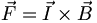$\vec F = \vec I \times \vec B\,$

You can easily find the direction of this force using the Right Hand Rule. The Right Hand Rule states that if you point your right hand fingers along the direction of current, I, and curl them towards the direction of the magnetic flux, B, the direction of force is along the thumb. See the picture below.

Now, imagine this single wire is replaced with a loop of wire. Between the magnets poles, this looks like two wires with current flowing in opposite directions. The forces on the wires cause the loop to rotate with forces as shown.

This coil is attached to the rotor and rotates. As it does so, the magnitude and direction of the force on the wires remains constant. However, the resultant torque varies with the angle. Look at the picture below. When the coil starts, there is maximum torque. As the coil moves, the moment arm is reduced and the torque decreases. Finally, when the coil is vertical (in the picture), there is no torque.

To keep a (almost) constant torque on the rotor, there are two things that happen. First, the current through the coil is reversed every half turn. So instead of an alternating torque like the one in the first figure below, the torque is always in the same direction. Also, additional coils can be used. When these coils are offset at different angles around the motor, the resultant torque becomes the sum of the colored torque curves in the figure below. The resultant torque is alway greater than zero, but is not constant. This variation is called torque ripple.

The process of switching current direction is called commutation. To switch the direction of curent, brushed DC motors use brushes and commutators. Commutation can also be done electronically (see Brushless DC Motors). The following diagram shows how brushes and commutators work.

## Equations

We start by writing the equation for conservation of energy in the motor. The power is input as electrical power,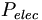$P_{elec}\,$ and the motor converts that to mechanical power,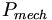$P_{mech}\,$. However, some of the power is lost as heat,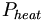$P_{heat}\,$.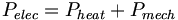$P_{elec}=P_{heat}+P_{mech}\,$

We can re-write this in terms of electrical and mechanical quantities as$iv=i^2R+\tau\omega\,$

The wire coils have both a resistance, R, and an inductance, L. When the motor is turning, the current is switching, causing a voltage,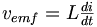$\begin{matrix}v_{emf} = L \frac{di}{dt}\end{matrix}$

This voltage is called the back-emf (electromotive force). The motor constants relate various quantities in a motor. They are a property of the design and material of each individual motor. The speed constant relates the back-emf to the output speed of the shaft,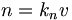$n=k_nv\,$

where n is the rotation in revolutions-per-minute (rpm). The torque constant relates the input current (in the windings) to the torque of the output,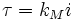$\tau = k_Mi\,$

These two constants are actually the same number with different units. The relationship between the two is$k_n \cdot k_M = \begin{matrix} \frac{30,000}{\pi} \end{matrix}\,$

Now look at the electrical side. If we sum the voltages, we get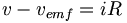$v-v_{emf}=iR\,$

This back-emf voltage is working against the voltage we apply across the terminals. Using the constants from above, we get this equation,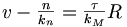$v-\begin{matrix}\frac{n}{k_n}\end{matrix}=\begin{matrix}\frac{\tau}{k_M}\end{matrix}R\,$

The maximum torque, or stall torque, is the torque at which n = 0 or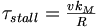$\tau_{stall} = \begin{matrix}\frac{vk_M}{R}\end{matrix}\,$

From this we can find the stall current, or starting current,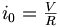$i_0 = \begin{matrix}\frac{V}{R}\end{matrix}\,$

The no-load speed is the maximum speed the motor can turn. To find its value, we set the torque equal to zero and we get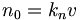$n_0 = k_nv\,$

This relationship means that, given a constant voltage, the motor will settle at a constant speed. This linear relationship produces the constant voltage speed-torque line$n = n_0 - \begin{matrix}\frac{n_0}{\tau_{stall}}\end{matrix}\tau\,$

The graph below is the dc motor speed-torque graph for a Maxon brushed DC motor. The dashed line represents the constant voltage speed-torque line.

Below is part of a datasheet for a series of Maxon brushed DC motors. This table gives the the numerical values for the motor properties and constants.

Line Number Name Symbol
3 No-load speed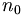$n_0\,$
4 Stall torque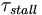$\tau_{stall}\,$
5 Speed/Torque Gradient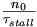$\begin{matrix}\frac{n_0}{\tau_{stall}}\end{matrix}\,$
7 Starting current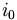$i_0\,$
8 Terminal Resistance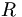$R\,$
14 Torque Constant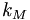$k_M\,$
15 Speed Constant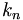$k_n\,$
18 Terminal Inductance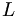$L\,$